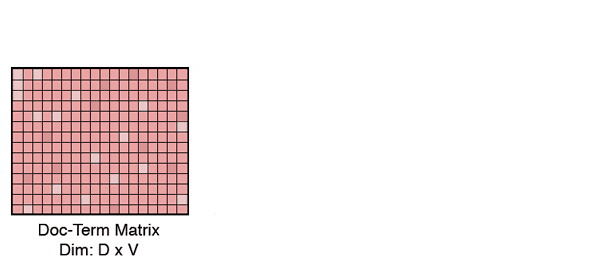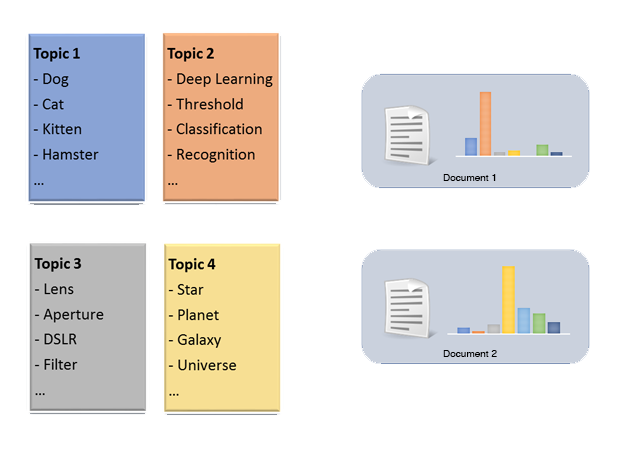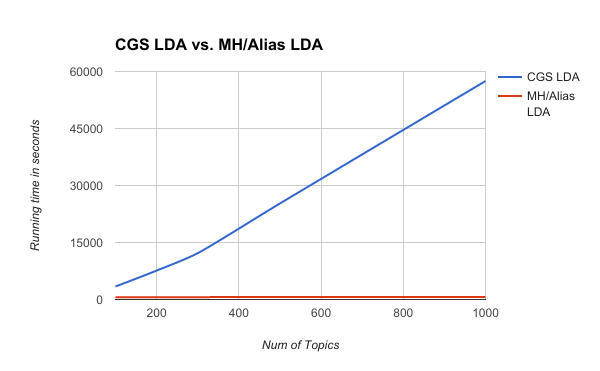# Clustering Similar Stories Using LDA

There is more to a story than meets the eye, and some stories deserve to be presented from more than just one perspective. With Flipboard 4.0, we have released story roundups, a new feature that adds coverage from multiple sources to a story and provides you with a fuller picture of an event.

Here’s how it looks:With our scale of millions of articles and constant stream of documents, it’s impossible to generate these roundups manually. So, we have developed a clustering algorithm that’s both fast and scalable, and in this blog post, I will explain how we create these roundups on Flipboard.

## Why is this difficult?

Although there are many sophisticated automatic clustering algorithms, such as K-means or Agglomerative clustering, story clustering is a non-trivial problem. Because each text document can contain any word from our vocabulary, most text document representations are extremely high-dimensional. In high-dimensional spaces, even basic clustering or similarity measures fail or are very slow.

Additionally, two very similar documents often have very different word usages. For example, one article may use the term kitten and another may use feline, but both articles could be referring to the same cat.

Furthermore, we don’t know the number of roundups that we expect to see beforehand. This makes it difficult for us to directly use parameteric algorithms such as K-means. Our clustering algorithm also needs to be fast and easy to update, because there is a constant stream of documents coming into our system.

## Overview

Since even the most basic distance measures fail in high dimensions, the first thing we do is lower the problem’s dimensionality. We represent each of our text documents as a bag-of-words, and remove stop-words and rare words from our vocabulary. Even after an aggressive trimming, the documents are still very high-dimensional. We then we use Latent Dirichlet Allocation (LDA) to further lower the documents’ dimensionality. We use LDA because this algorithm is amenable for text modeling and provides us with interpretable lower dimensional representations of documents.

LDA is generally used for qualitative understanding of big text corpora. The latent topics that the model learns are highly interpretable and provide deep insights to the data. However, that’s not the only thing it can be used for; it is also a very logical algorithm to use for dimensionality reduction as it learns a mapping of sparse document-term vectors to sparse document-topic vectors in an unsupervised setting.Once the documents are represented over a tractable number of dimensions, all similarity and distance measures come into play. For our story clustering, we simply map all the documents to this conceptual level (latent topics), and look at the neighbours for each document within a certain distance. We use an approximate nearest neighbour model because it only requires us to look at a small neighbourhood of documents to generate these clusters.

The following sections explain how we use LDA for this problem, and then introduce some tricks to optimize LDA using Alias tables and Metropolis-Hastings tests.

## High-level overview of LDA

LDA is a probabilistic generative model that extracts the thematic structure in a big document collection. The model assumes that every topic is a distribution of words in the vocabulary, and every document (described over the same vocabulary) is a distribution of a small subset of these topics. This is a statistical way to say that each topic (e.g. space) has some representative words (star, planet, etc.), and each document is only about a handful of these topics.For example, let’s assume that we have a few topics as shown in the figure above. Knowing these topics, when we see a document explaining Detecting and classifying pets using deep learning, we can confidently say that the document is mostly about Topic 2 and a little about Topic 1 but not at all about Topic 3 and Topic 4.

LDA automatically infers these topics given a large collection of documents and expresses those (and future) documents in terms of topics instead of raw terms. The key advantage of doing this is that we allow term variability as the document is represented at a higher conceptual (topic) level rather than at the raw word level. This is akin to many success stories in image classification tasks using deep learning, where the classification is done on a higher conceptual level instead of on the pixel level.

To infer the above latent topics, we do posterior inference on the model using Gibbs Sampling. What it essentially comes down to is to estimate the best topic for each word seen in every document. This is estimated by:

where $w$ is the word seen in the $n$th position in document $d$, $C_k^d$ is the number of times the topic $k$ has appeared in document $d$, $C_k^w$ is the number of times the word $w$ has been estimated with topic $k$ in the whole corpus, and $C_k$ is the number of times the topic $k$ has been assigned in the corpus. It is a sensible equation which suggests that a topic is more likely to be assigned if it has been assigned to other words in the same document or when the term has been assigned to that same topic several times in the whole document corpus.

We calculate the above equation for each topic and define a multinomial distribution (a weighted dice roll), and generate a random topic from that distribution. The code for LDA’s inference (CGS-LDA) looks like this:

1
2
3
4
5
6
7
8
9
10
11
12
for (d = 0; d < D; d++) {
for (n = 0; n < len(data[d]); n++) {
w = data[d][n];
k = Z[d][n];
decrement_count_matrices(d, w, k);
for (k = 0; k < K; k++) {
p[k] = (CDK[d][k] + alpha) * (CWK[w][k] + beta) / (CK[k] + V * beta);
}
k = multinomial_distribution(p);
increment_count_matrices(d, w, k);
}
}


This computation can be very expensive if we try to capture more than a thousand topics, because the algorithmic complexity becomes $O(DNK)$ per iteration. What we would ideally like is to get rid of $O(K)$ loop for each word in a document.

## Optimizing LDA using Alias Tables and Metropolis-Hastings Tests

Fortunately, there has been a lot of new research (LightLDA, AliasLDA) to speed up the sampling process and reduce the computational complexity to $O(DN)$. The key question here is: Is it really possible to generate a single sample from a weighted multinomial distribution in under $O(K)$ time?

The answer, not surprisingly, is “no” because generating a $K$ dimensional multinomial probability array $p$ takes at least $O(K)$ time because we need to know the weight for each index. But once this array is created, generating a sample is simply a matter of generating a random number from $[0, sum(p)]$ and checking which index of the array the number falls in. And if we needed more samples from the same distribution, all future samples would only require $O(1)$ time.Instead of taking a single sample each time from the distribution, if we take $K$ samples each time the table is generated, then the amortized sampling complexity would be $O(1)$. But this method has huge memory implications because the sizes of these arrays are dependent on the sum of the weights, and in LDA’s case, they can get extremely massive due to dependencies on count matrices ($C_d^k$, $C_k^w$ and $C_k$).

### Alias Sampling

Walker’s Alias method is an effective way to compactly store these probability vectors (Space complexity: $O(K)$), while keeping the sampling complexity at $O(1)$. Instead of defining a long row vector, Alias sampling defines a completely filled 2-dimensional table (dim: $K \times 2)$ from which its easy to sample from in constant time.

These tables are generated by first multiplying each element by $K$, followed by a Robin Hood algorithm and maintaining two lists: rich and poor. rich contains all the elements that have a greater weight than $1.0$, and the poor stores the rest. This is followed by a simple iteration of putting the poor elements in the table cell first, and then filling up the height if needed by “stealing” from the rich:

Each column has a height of 1.0 with a maximum of two different array indices. For sample generation, a random number is generated between $[0, len(table)]$ to pick the column, and then we generate a random floating point between $[0, 1.0]$ and see which region the decimal number falls in.

### Metropolis Hastings Algorithm / Test

Coming back to our LDA case, every time we need to sample a topic for a word in a document, we could generate $K$ samples. However, that would be wrong because the update equation is dependent on the count matrices, and stale samples don’t represent the true probability distribution of the inference.

Here’s where Metropolis-Hastings (MH) algorithm comes into play. MH algorithm is a Markov-Chain Monte Carlo (MCMC) method to move around the probability space with the intention of converging to an objective. Given an objective function $O(\cdot)$, a proposal function $P(\cdot)$ and a proposed position in the probability space, MH algorithm acts as a guide telling the algorithm whether it is a good or bad idea to move from the current position $x$ to the proposed point $x'$. The acceptance of a new proposed position is computed by:

If the algorithm thinks that it’s a great idea to move, MH will always accept the proposal.

The following toy example shows how we can approximate the area of a random function with Metropolis-Hastings sampling. In this example, we try to approximate the shape of a complicated non-linear function and use a standard Gaussian distribution ($\mathcal{N}(0, 1)$) multiplied with a step-size to generate proposals.

From the above example, it can be seen that proposal functions control the convergence speed of the algorithm. In the ideal scenario, we would like a high-acceptance rate and the ability to move quickly around the space. For the toy example above, step-sizes of $0.2 - 0.4$ achieve the best results.

### Back to LDA

We would like to have high proposal acceptance rates, good space coverage, and simple proposal generation complexity. LightLDA authors suggest using the expressions within the LDA’s update equation as proposal functions. These expressions match the function in certain regions. This has two further advantages: we don’t need to compute anything extra and simply use the statistics (count matrices) that we would have collected anyway, and we don’t actually need to create alias tables for one of the proposals.

$Z_d$ acts as a proxy alias table for the doc-proposal because it stores the number of times each topic has appeared in the document $d$, and if we generate a random number between $[0, len(d)]$, we get a sample from the doc-proposal. For the word-proposals, we generate alias tables for each word and use the afforementioned Alias Sampling trick.

We cycle between these two proposals, and do the MH test on the fly, and accept/reject the proposals. We recompute an alias table for a word every time we have used $K$ proposals. The acceptance probabilities for doc-proposal ($A_d$) and word-proposal ($A_w$) given a proposal topic $p$ and the current topic $k$ can be calculated by using the LDA’s update equation as the objective function, and the doc and word proposals as the proposal functions.

Using Alias tables and MH tests, the algorithm looks like the following:

1
2
3
4
5
6
7
8
9
10
11
12
13
14
15
16
17
18
19
20
21
22
23
24
25
for (d = 0; d < D; d++) {
for (n = 0; n < len(data[d]); n++) {
proposal = coinflip();
w = data[d][n];
k = Z[d][n];
decrement_count_matrices(d, w, k);
if (proposal == 0) {
// doc-proposal
index = randomInt(0, len(data[d]));
p = Z[d][index];
mh_acceptance = compute_doc_acceptance(k, p);
} else {
// term-proposal
p = alias_sample(w);
mh_acceptance = compute_term_acceptance(w, p);
}
// MH-test
mh_sample = randomFloat(0, 1);
if (mh_sample < mh_acceptance) {
increment_count_matrices(d, w, k);  // reject proposal, revert to k
} else {
increment_count_matrices(d, w, p);  // accept proposal
}
}
}


By doing this, the algorithmic complexity of LDA comes down to an amortized $O(DN)$ which allows us to process documents an order of magnitude faster. The following table and the graph compare the runtime (in seconds) of LDA of 60,000 documents after 100 iterations (convergence) on a single process.

Num. of Topics 100 200 300 500 1000
CGS LDA 3427.99 7605.02 12190.54 25274.20 57492.22
MH/Alias LDA 601.06 616.07 620.82 646.38 685.19## Clustering

LDA provides us with a sparse and robust representation of texts that reduces term variability in much lower dimensions. In 1000 or lower dimensions, most simple algorithms work really well. When each document is represented in this space, we do a fast Approximate Nearest Neighbour search, and cluster all documents that are within a certain distance from each other.

Using a distance based metric has an added advantage of being able to capture near and exact duplicates. The documents that are mapped too close to each other (purple circle) are considered to be the same story. The documents that are a certain radius away from the exact duplicates (pink circle) make up the roundups for each story.

Removing exact duplicates helps in capturing different views on an event, and here is an example of one of our story-clusters where the roundups capture differing perspectives on the same news.## Conclusion

Story roundups directly help us in diversifying our users’ feeds while also providing users with multiple perspectives on important stories. We had a lot of fun implementing this cool new feature, not least because we came across several new tricks that can be applied in multiple domains.

Alias Sampling and MH algorithm have been around for a long time but they are only now being used in tandem to optimize posterior and predictive inference problems.

### Key Takeaways

• LDA is awesome not just in context of qualitative topic analysis but also in terms of dimensionality reduction.
• Simple algorithms work really well in low dimensions; (almost) everything fails in very high dimensions.
• Bayesian Machine Learning doesn’t have to be slow or expensive.
• If you made it this far, I hope you learned a new way to optimize some posterior inference problems - Alias Tables and MH tests are an amazing combination.

Extra special thanks to Ben Frederickson for suggestions, edits and the Alias Table visualization. Thanks to Dale Cieslak, Mike Vlad Cora and Mia Quagliarello for proofreading.

Enjoyed this post? We’re hiring!